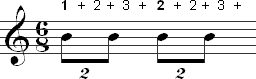##### What You Will Learn
1. How compound time signatures are different from simple time signatures
2. What the numbers in a compound time signature mean
3. Counting eighth and sixteenth notes
4. Counting duplets in compound time signatures

# Counting Rhythms in Compound Time Signatures

## The Difference Between Simple and Compound Time Signatures

### Subdivision of the Beat

All of the rhythms you have learned so far have been in simple time signatures. A simple time signature subdivides each beat into two parts. It is also possible to have a time signature where each beat is subdivided into three parts. These are known as compound time signatures. 6/8, 9/2, and 12/16 are examples of compound time signatures.

### What the Numbers Mean

In a simple time signature, the top number gives the number of beats per measure, while the bottom number tells you which kind of note gets the beat. Compound time signatures don't work this way. The meaning of the numbers in a compound time signature are explained below.

#### The Top Number

The top number represents the number of subdivisions in a measure. For example, if the subdivision is an eighth note and the top number is six, there would be six eighth notes per measure. Don't confuse this with the beat. The top number in a compound time signature must be divided by three in order to determine the number of beats per measure. Dividing the '6' in 6/8 by three equals two. This means that there are two beats in each measure of 6/8.

#### The Bottom Number

The bottom number represents the note value that subdivides each beat. This number can be used to determine the kind of note that gets the beat by adding three notes of this value together. The resulting note will always be a dotted note of some kind. For example, the bottom number in 6/8 time is '8', which represents an eighth note. If you add three eighth notes together, they equal a dotted quarter note. The dotted quarter note is the note that gets the beat in a measure of 6/8 time.

If you combine what you learned from each of these numbers, you find that 6/8 means that there are two beats per measure with the dotted quarter note getting the beat.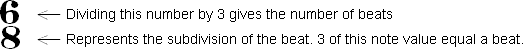### How to Tell Simple and Compound Time Signatures Apart.

It is relatively easy to distinguish between simple and compound time signatures. A time signature is compound if the top number is greater than three and can be divided by three. Any time signature where the top number is equal to three or is another number that can't be divided by three is simple. This is a bit of an oversimplification since composers may occasionally group rhythms in a time signature that is normally compound in such a way that it is no longer compound. However, most music doesn't do this and the rule given above will work in most cases.

### Summary of Differences Between Simple and Compound

The chart below summarizes the differences between simple and compound time signatures.

Characteristic Simple Compound
Top number in time signature Gives the number of beats per measure Dividing the number by 3 gives the number of beats per measure. If the number is greater than 3 and is also divisible by 3, the time signature is probably compound.
Bottom number in time signature Indicates the type of note that gets the beat. Indicates the note value for the subdivision of the beat. Adding 3 of this value together gives a dotted note value equal to one beat.
Subdivision Beat is subdivided into two parts. Beat is subdivided into three parts.
The beat A normal note (quarter note, half note, etc.) A dotted note value (dotted quarter note, dotted eighth note, etc.)

## Common Compound Time Signatures

The chart below shows some frequently used compound time signatures with details on how many beats per measure and which note gets the beat.

Time Signature Number of Beats Type of Note that Gets the Beat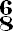2 dotted quarter note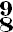3 dotted quarter note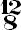4 dotted quarter note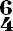2 dotted half note

## Equivalent Time Signatures

Compound signatures have variations that are equivalent to each other just like simple time signatures. Some time signatures are more common than others, but variations do occur. The most common compound time signatures are 6/8, 9/8, and 12/8. However, you will also see time signatures like 6/4, 12/16, and 9/2 from time to time.

## Triplets and Compound Time Signatures

Many people confuse compound time signatures and triplets. It is incorrect to refer to the subdivision in a compound time signature as a triplet. Remember that a triplet is three notes of one value in the space of two notes of the same value. Since the normal subdivision in a compound time signature is three, it can't be a triplet because the subdivision occurs in the space of three notes, not two.

It is possible to rewrite music in a compound time signature by using triplets in a simple time signature. Here is an example of the same passage notated in a simple time signature using triplets: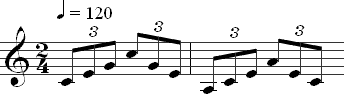This passage is rewritten in a compound time signature below.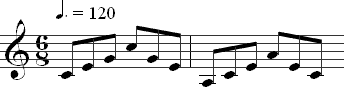## Subdivisions in Compound Time Signatures

After the first subdivision of the beat, the rhythms in a compound time signature are subdivided pretty much like rhythms in simple time signatures. For example, in 6/8, the dotted quarter note is subdivided into three eighth notes. Each eighth note is subdivided into two sixteenth notes. Sixteenth notes are subdivided into thirty-second notes. Below is a chart that shows how dotted quarter notes are subdivided into eighths and then sixteenths in 6/8: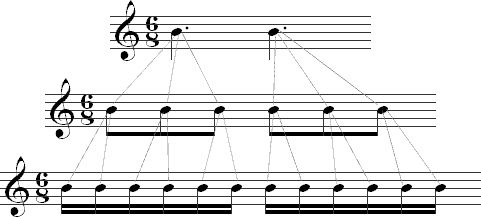## Counting in Compound Time Signatures

Rhythms consisting of notes values equal to or greater than a beat can be counted as you would any other time signature. The chart below shows how this works in 6/8, 9/8, and 12/8.

Type of Time Signature Example Time Signatures How to Count Musical Example
Duple 6/8, 6/4, 6/2 Count: one, two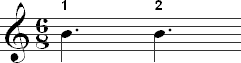Triple 9/8, 9/4, 9/2 Count: one, two, three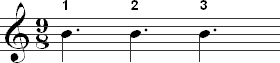Quadruple 12/8, 12/4, 12/2 Count: one, two, three, four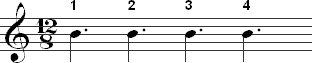### Counting Eighth Notes in Compound Time Signatures

The chart below shows two methods for counting eighth notes in compound time signatures. 12/8 is used for the examples, but the methods can easily be adapted to any other compound time signature.

Count Example Notes
• one two three,
• two two three,
• three two three,
• four two three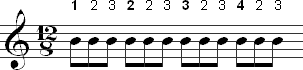This method is somewhat awkward at first, but it is a good approach because it is easy to keep track of beats and count subdivisions beyond an eighth note.
• one and a,
• two and a,
• three and a,
• four and a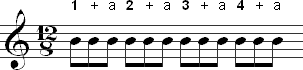This is a simple method that works well with music that includes only one subdivision of the beat, such as an eighth note in 12/8. It doesn't work as well with subdivisions beyond this.

### Counting Sixteenth Notes in Compound Time Signatures

Sixteenth notes can be counted by adding "and" between each number. This is similar to how eighth notes are counted in simple time signatures. Using this approach for 6/8, would result in the count "one and two and three and, two and two and three and." This is shown below: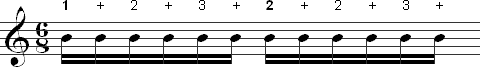### Counting Duplets

A duplet is a common rhythm in compound time signatures. Like a triplet, it is a tuplet. A duplet is when two notes of one value occur in the space of three notes of the same value. It is indicated with a "2" above or below the two notes. In a compound time signature such as 6/8, this means that an eighth note duplet is two eighth notes that occur in the space of three eighth notes. The use of duplets can make a compound time signature feel like it is a simple time signature because the characteristic feel of the compound time signature is lost when the beat is no longer subdivided by three. Duplets can be counted by keeping track of the sixteenth notes. The first duplet occurs on the beat. The second one occurs on the "and" of two. Below is an example of duplets in 6/8 with the count shown above the music: